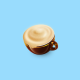# Triangles

### 5 guesses to Triangles

Warning: Use of undefined constant bfa_comments - assumed 'bfa_comments' (this will throw an Error in a future version of PHP) in /home/customer/www/riddledude.com/public_html/wp-content/themes/atahualpa/comments.php on line 132
•MMG

•Dude

FOUR is correct, MMG, but can you tell us why?

•MMG

Dear, Riddle Dude(S)

Because if you add 4 to all the number on the two other sides of one of the triangles they equal to the square of the number in the middle of the circle.Here is all the calculation to clarify what I mean.

4+5+16=25
Therefore:
5*5=25

4+2+3=9
Therefore:
3*3=9

8+4+4=16
Therefore:
4*4=16

These are all my calculations.

P.S I really like this website and have spread it to people in my school. I hope you post some more mathematical problems as I enjoy numbers

Thanks, MMG

•Dude

Absolutely correct, MMG.

You are today’s winner.

•Bunion